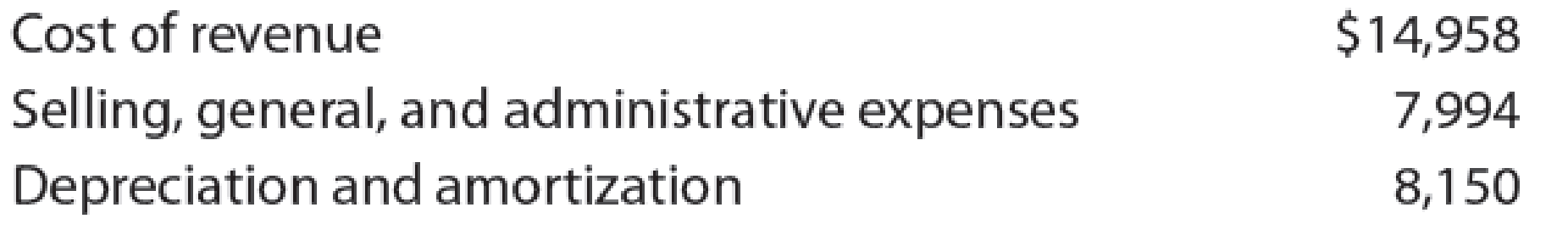# Break-even analysis for a service company3 Sprint Corporation (S) is one of the largest digital wireless service providers in the United States. In a recent year, it had approximately 60 million direct subscribers (accounts) that generated revenue of $33,347 million. Costs and expenses for the year were as follows (in millions): Assume that 30% of the cost of revenue and 70% of the selling, general, and administrative expenses are fixed to the number of direct subscribers (accounts). a. What is Sprint’s break-even number of accounts, using the data and assumptions given? Round to one decimal place. b. How much revenue per account would be sufficient for Sprint to break even if the number of accounts remained constant? Round to one decimal place.BuyFindarrow_forward ### Financial And Managerial Accounting 15th Edition WARREN + 1 other Publisher: Cengage Learning, ISBN: 9781337902663 #### Solutions Chapter SectionBuyFindarrow_forward ### Financial And Managerial Accounting 15th Edition WARREN + 1 other Publisher: Cengage Learning, ISBN: 9781337902663 Chapter 20, Problem 16E Textbook Problem 1 views ## Break-even analysis for a service company3Sprint Corporation (S) is one of the largest digital wireless service providers in the United States. In a recent year, it had approximately 60 million direct subscribers (accounts) that generated revenue of$33,347 million. Costs and expenses for the year were as follows (in millions):Assume that 30% of the cost of revenue and 70% of the selling, general, and administrative expenses are fixed to the number of direct subscribers (accounts). a. What is Sprint’s break-even number of accounts, using the data and assumptions given? Round to one decimal place. b. How much revenue per account would be sufficient for Sprint to break even if the number of accounts remained constant? Round to one decimal place.

a.

To determine

Compute Company SN’s break-even number of accounts.

### Explanation of Solution

Break-even Analysis: It refers to an analysis of the level of operations at which a company experiences its revenues generated is equal to its costs incurred. Thus, when a company reaches at its break-even, it reports neither an income nor a loss from operations. The formula to calculate the break-even point in sales units is as follows:

Break-evenpointinSales(units) =FixedCostsContributionMarginperunit

Compute the Company SN’s break-even number of accounts.

Break-evenpointinSales(units) =FixedCostsContributionMarginperunit=$18,233.2million(1)$341.3peraccount(2)=53.4millionaccounts

Working note (1):

Determine the total fixed cost.

 Particulars Total costs (in millions) (A) Fixed cost percentage (B) Fixed costs (in millions)  (A×B) Cost of revenue $14,958 30%$4,487.4 Selling, general, administration expense $7,994 70%$5,595.8 Depreciation $8,150 100%$8,150.0 Total fixed costs \$18,233.2

Table (1)

Working note (2):

Calculate the contribution margin per account

b.

To determine

Compute the revenue per account for break-even, if the number of accounts remains constant.

### Still sussing out bartleby?

Check out a sample textbook solution.

See a sample solution

#### The Solution to Your Study Problems

Bartleby provides explanations to thousands of textbook problems written by our experts, many with advanced degrees!

Get Started

Find more solutions based on key concepts
What are the disadvantages of a corporation?

Foundations of Business (MindTap Course List)

Why is productivity important?

Principles of Economics (MindTap Course List)

What is the role of network administration?

Accounting Information Systems

Calculate the missing items in the following:

College Accounting (Book Only): A Career Approach

Should stockholder wealth maximization be thought of as a long-term or a short-term goal? For example, if one a...

Fundamentals of Financial Management, Concise Edition (with Thomson ONE - Business School Edition, 1 term (6 months) Printed Access Card) (MindTap Course List)

Explain why it is easy to update a TDABC model.

Cornerstones of Cost Management (Cornerstones Series)